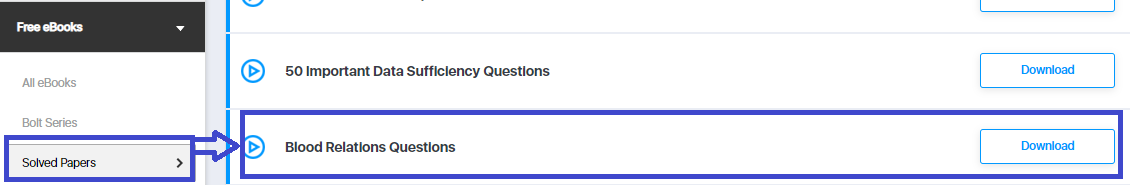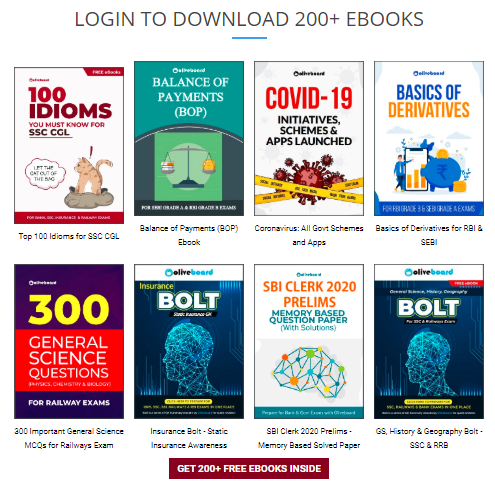# 50 Important Blood Relation Questions PDF For Practice | Download Here

Blood Relations is one of the most studied topics of the Logical Reasoning section and is one of the important for every banking and government entrance exam. This topic tests the analytical skills of students and how students can approach the solution for the questions asked with the help of a diagram instead of calculations. In this blog, we have brought you a free ebook on 50 Important Blood Relation Questions for practice.

Download the free ebook here and practice all the important questions here.

Want to attempt a free mock test? Take A Free Mock Test Here

## 50 Important Blood Relation Questions PDF | Free Ebook

Click on the link provided below and get the complete free PDF.Step 1: Click on the above-given download link. You will be taken to Oliveboard’s FREE Ebooks Page.Step 2: Register/Login on to the Free E-Books Page of Oliveboard (It is 100% free, You just enter your valid email id and a password to be able to download the Important Blood Relations Questions & Answers).

Step 3: After Logging in, you will see the list of Free Ebooks.

Step 4: Click on the Solved Papers section under All ebooks sections.## Here’s A Sneek Peek Into Ebook

Directions (1-3): Read the following information carefully and answer the questions given below.

A+B means A is the daughter of B.
A-B means A is the husband of B.
A*B means A is the brother of B.

Q1) If P+Q-R, which of the following is true?

1) R is the mother of P
2) R is the sister in law of P
3) R is the aunt of P
4) None of these

Q2) If P*Q+R, which of the following is true?

1) P is the brother of R
2) P is the uncle of R
3) P is the son of R
4) None of these

Want to attempt a free mock test? Take A Free Mock Test Here

Q3) If P+Q*R, which of the following is true?

1) P is the niece of R
2) P is the daughter of P
3) P is the cousin of R
4) P is the daughter in law of R

Q4) If A \$ B means “A is the father of B”, A # B means ‘A is the daughter of B’, A @ B means
‘A is the sister of B’, then how is K related to M in H @ K \$ L # M?

1) Husband
2) Uncle
3) Father
4) Cannot be determinedOliveboard Bolt series E-books: